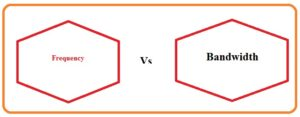Hello guys, I hope you all are doing great. In today’s tutorial, we will have a look at Difference Between Frequency and Bandwidth. In data transmission there two most commonly used terms first is the frequency and the second one is bandwidth.  frequency explained the number of completion of cycles in unit time. While bandwidth is the transfer of data in unit.

The unit for both these terms is the same which is hertz. Both have different features and parameters. In today’s post, we will have a detailed look at their comparison and find their differences. So let’s get started with Difference Between Bandwidth and Frequency.

#### Difference Between Bandwidth and Frequency

Frequency

• Frequency is the number of complete cycles in unit time.
• The completed cycles of wave in unit time are called frequency.
• The time though which cycle is repeated is called period it is the inverse of frequency
• It used to discuss the rate of oscillation and vibration in different scientific fields.
• The frequency of ac system is sixty or fifty hertz according to our country
• The range of frequency that can be heard is fifteen hertz to twenty-kilo hertz
• Radiofrequency has thirty to three hundred hertz
• One-half cycle called alternation
• It denoted as f’.
• F=I/t
• It measured in hertz.
• It employed for observation of light frequency in different aspect
• Frequency also called the inverse of the time period.
• One hertz is equal to the complete rotation in one second.
• One complete waveform of the current is called a cycle
• It formula is f=v/λ.
• The sound of frequency which we can lies between twenty hertz to twenty-kilo hertz.

Bandwidth

• Bandwidth explains the transmission of data in unit time.
• Different test like TCP PRTG are used to measure the value of bandwidth
• Bandwidth is measured in different units like Mbps, Tbps, Gbps, Tbps
• It can be compared to the water flow in the pipe the quantity of water flow is called bandwidth
• It employed in different types of fields of education in signal processing used to finds the value of high and low frequency.
• It is defined in terms of bit rate and calculates din bps.
• It used to define the capacity of the link and the main fact to define the calculation of quality speed on the net connection
• It is the larges data transmission over a single place.
• It can be defined in terms of data or digital bandwidth
• It is contrary to the signal bandwidth used to measure frequency.
• It defines in terms of bits per second.
• It denoted as B.
• Its unit is hertz.### Frequency vs Bandwidth

Frequency Bandwidth
It defines as the number of cycles in one second of a periodic signal it is known as the range of frequency over which a signal is transmitted
It measuring unit is Hertz (Hz) It measured in Hertz (Hz)
It finds the pitch of sound waves and light waves’ color It is used for measuring the information details carried through signal
It used to carry info from one point to another like radio TV or wifi signals It denotes how much data can be transmitted in a given period
it is a measure of the rate at which the signal repeated it is a calculation of frequency ranges that a signal can carry
It is low or high Its shape can be narrow or wide
It is a basic property of period wave It is a derived feature of the signal
It helps to differentiate between two signals through frequency difference it differentiates two signals through differnt bandwidths
It affects the propagation of signals through media like wire or the atmosphere It has effects on speed where data can be transmitted over a communication channel
It is used for tuning the radio to a certain station It is used for frequency band allocation to differnt communication systems
it is used to modulate carrier signals for carrying information it is used to define the spectral efficiency of a communication network
It can be affected by interference from another signal with the same frequency It can affect other signals with overlapping bandwidths
It is related to the waveform period it has a relation with the rate at which data is transmitted over a communication channel
Its representation signal is a sine wave it is represented through the spectrum of frequency
it measures the fundamental frequency component of the signal it is the measurement of the total frequency range used by the signal
it is used for the identification of the existence of signals in noisy conditions it used for the optimization of frequency bands
It used for the calculation of resonant frequency it is used for designing and optimizing communication system
it is a scalar quantity it Is a vector quantity
It used in different audio radar and wireless communication systems its main applications are modulation,  signal processing, and channel coding

### What is an example of frequency and bandwidth?

• Bandwidth is frequency bandwidth. The width is the highest frequency subtraction of the lowest frequency. Here is an example of hearing
• The person’s ear’s bandwidth is about 20000 hz – 20 hert= 19980 hertz

What is the difference between bandwidth and natural frequency?

• The natural frequency is the frequency where the response is at peaks. Bandwidth is known as the width of a peak at three decibels below peak response value

That is a detailed post about the Difference Between Frequency and Bandwidth